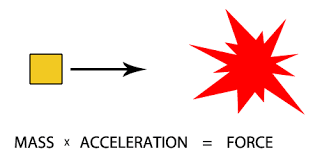# MCAT & Medical School AdmissionsThe Chemical and Physical Foundations of Biological Systems section of the Medical College Admissions Test (MCAT) is conceptually one of the most difficult sections of the exam. With 59 questions in a 95-minute span, it integrates and tests dense subjects such as biochemistry, biology, general chemistry, organic chemistry, and physics. This section not only requires a conceptual understanding of these topics, but also tests the student’s ability to interpret, analyze, and calculate the answer to complex problems. Because of this, it is vital that students know many equations cold prior to entering the test. Here we will provide you with recommendations for creating equation sheets and methods for memorizing them.

To best equip yourself for test day, it is essential that you have a reliable list of equations. Many students choose to compile their own during the content review portion of their study schedule. Because the section is highly integrated, this allows students to organize the equations in a way that makes the most sense to them and allows them to group and memorize these equations. If you are looking for a starting point for an equation sheet, Free MCAT Prep and Gold Standard have many of the necessary equations listed. Keep in mind that some students will need more equations explicitly written down so make sure to compare these lists to your primary content review resource so that you are not missing any equations you may personally need to commit to memory.

Now that you have started compiling your equation list, your next step is to begin memorizing. This is a daunting task for most students, and I can tell you that mass memorization of equations will follow you in medical school when you take courses like pharmacokinetics. Because of this, it is extremely helpful to equip yourself with methods for memorizing large lists and blocks of information such as this. While there are many memorization tools for this, we will focus on two methods for memorizing equations: chunking and relationships.

Chunking is arguably the most common method for memorizing these equations. The key is to smart with small chunks of information and then build on your knowledge. Begin by memorizing, for example, the equations for kinematics in physics. Get a blank sheet of paper out and write ‘kinematics’ at the top and number the page with the number of equations you are required to know for kinematics. Then set a timer for 30 seconds to a minute and write as many of the equations as you can. When the timer runs out, pull out your formula sheet and write down the formulas you forgot or wrote incorrectly in red on your practice sheet. Repeat this process until you can write your kinematics formulas without any corrections. And as you learn each section of formulas, you begin testing sections together in the same practice until eventually you are reproducing your entire formula sheet from memory. A couple of quick tips on reproducing the entire formula sheet: it helps to have the categories memorized in order and the number of required formulas that correspond to these categories. Often students create their own mnemonic to help with this or develop a method of reciting the categories and numbers to themselves. This process can be done in a week or two weeks but is best done over the entire course of your test preparation if you have time.Focusing on relationships between equations can also greatly improve your conceptual understanding of the relationships between physics, chemistry, organic chemistry and biochemistry. This is what defines the high scorers on this section of the MCAT. Rather than just ‘plugging and chugging’, this requires you to understand the units and relationships between the variables in the equations and how you can utilize multiple equations to get to your desired result. This becomes especially important in the electrical section of physics where there are many shared variables between equations and you can often manipulate multiple equations to produce other equations you have memorized. As you memorize equations, try to focus on what happens, for example, to acceleration if you increase mass. If the force is constant, we know that the acceleration must decrease linearly if we increase mass (F=ma). While this seems simple, it becomes more complex in later sections of physics and chemistry. Finally, understanding how to manipulate units to get desired units is extremely helpful in deriving forgotten equations with even just a conceptual understanding of this section.

With these methods, this should help you overcome the daunting task of memorizing pages of equations! The time and effort you put toward studying these equations will result in confidence on test day and hopefully produce great increases in your score. Best of luck!

#### About the Author

Jordan Salley is one of MyGuru's most accomplished MCAT tutors. Click here to learn more!